# Selina Solutions Concise Mathematics Class 6 Chapter 16 Percent (Percentage) Exercise 16(B)

Selina Solutions Concise Mathematics Class 6 Chapter 16 Percent (Percentage) Exercise 16(B) provides answers to the exercise wise problems in a comprehensive manner. Solutions are prepared by the experts with the intention to help students ace the examination. Detailed solutions help students clear their confusion about the concept that arises while solving the problems. To evaluate academic performance, students can refer to Selina Solutions Concise Mathematics Class 6 Chapter 16 Exercise 16(B) PDF, from the links mentioned below.

## Selina Solutions Concise Mathematics Class 6 Chapter 16: Percent (Percentage) Exercise 16(B) Download PDF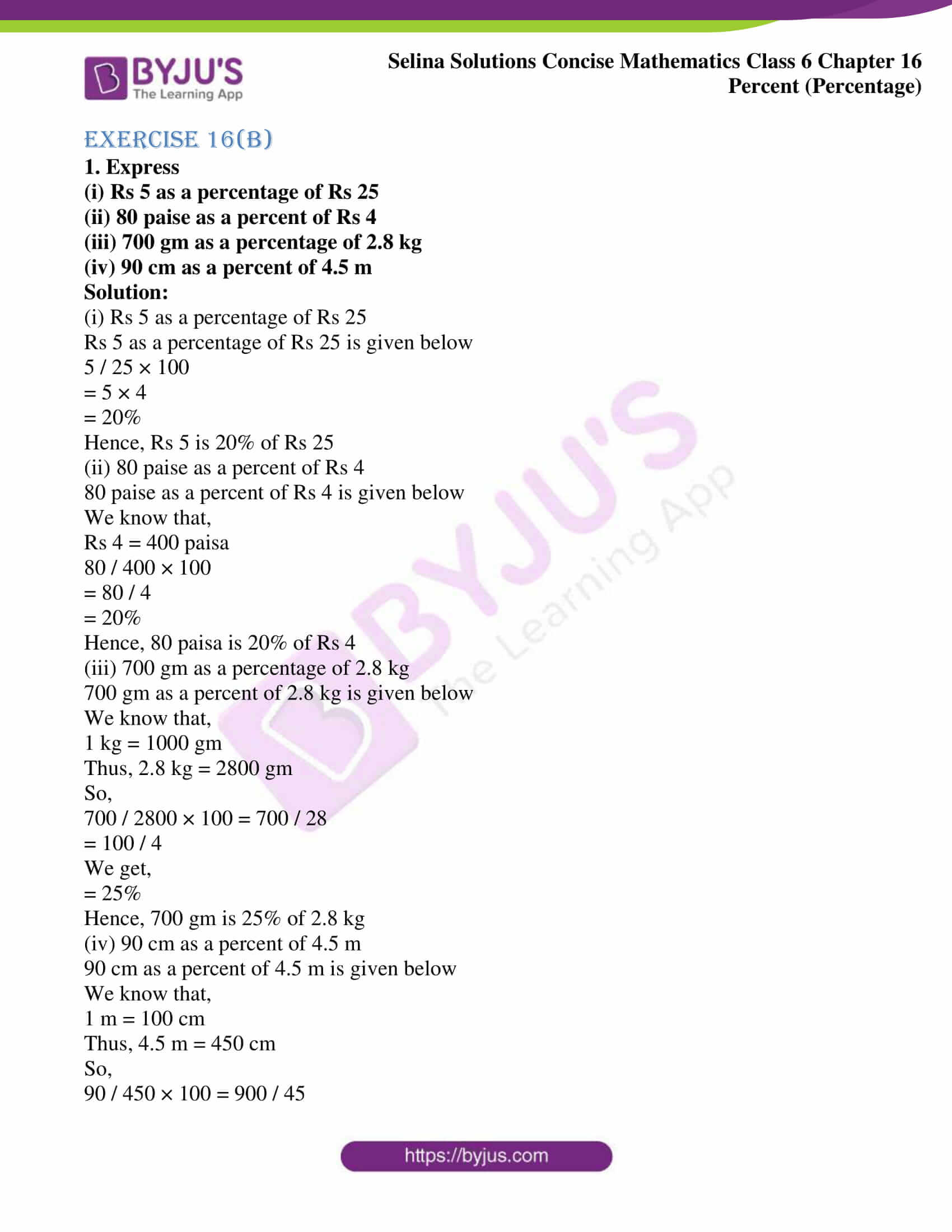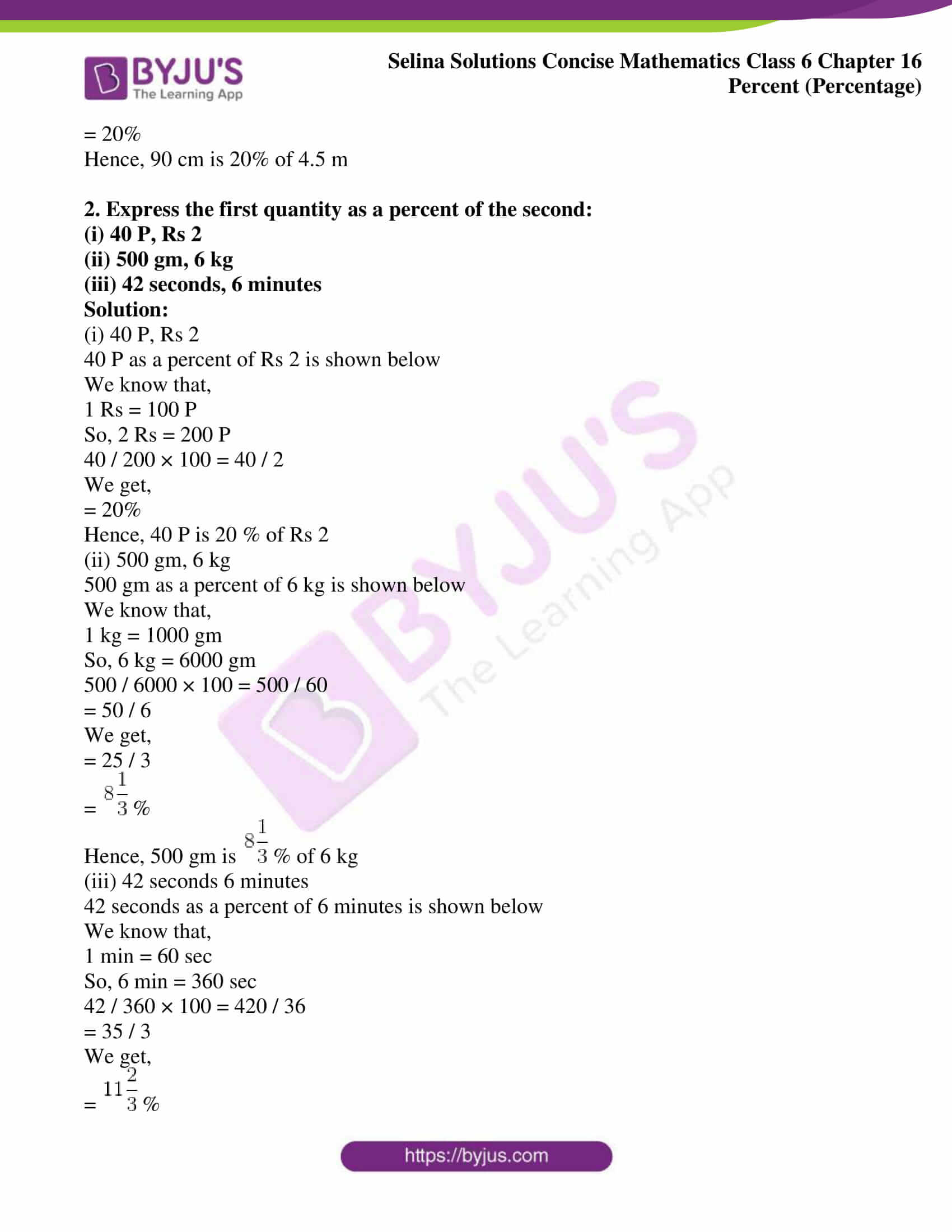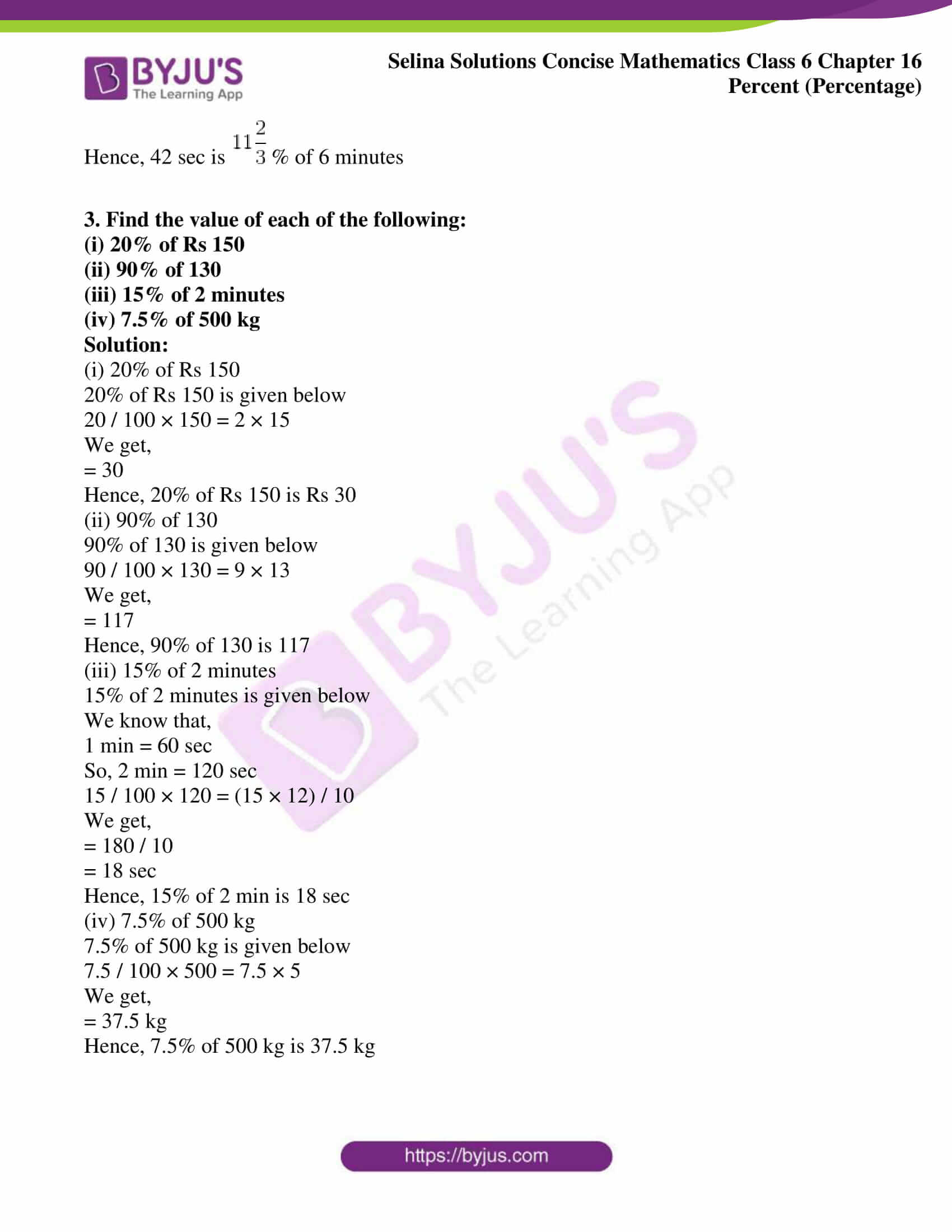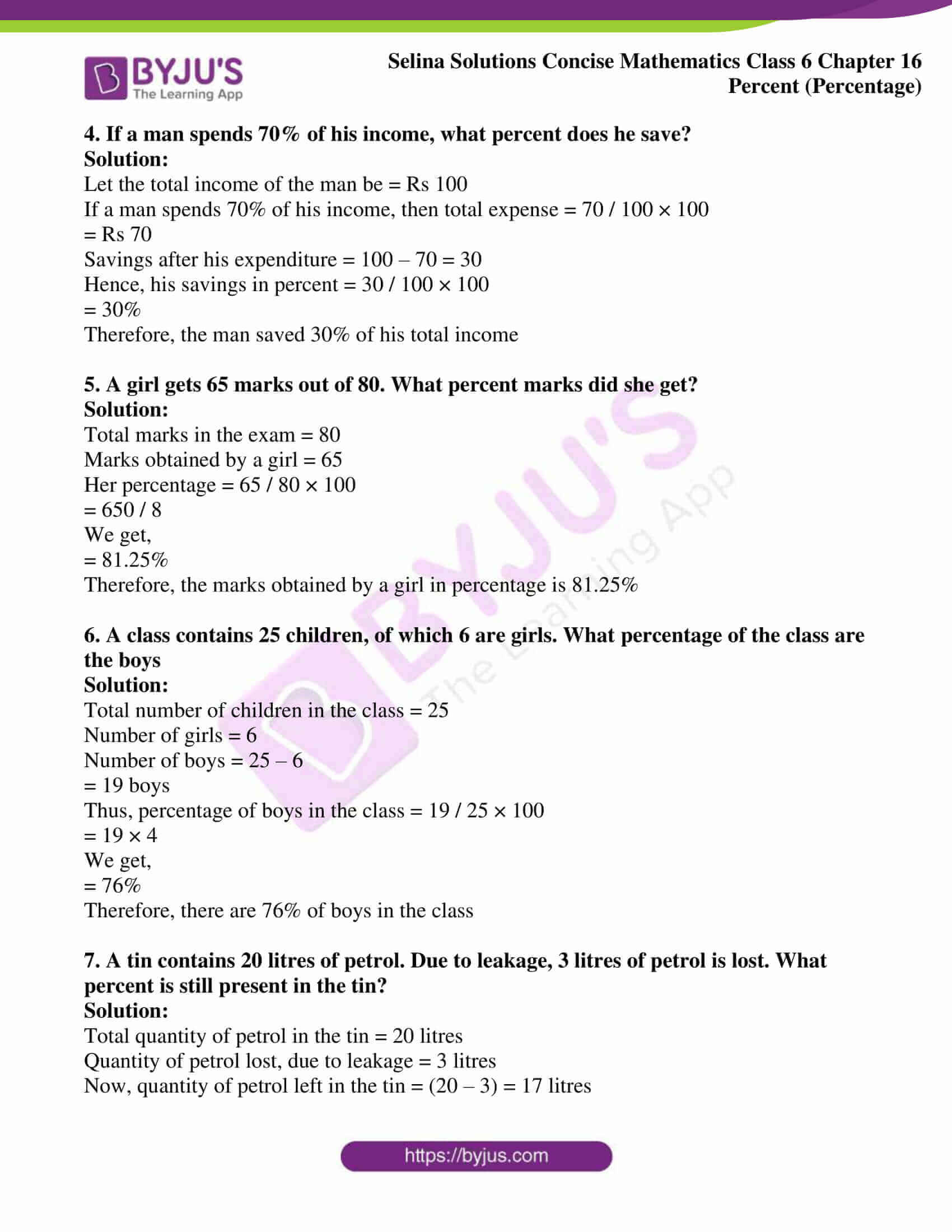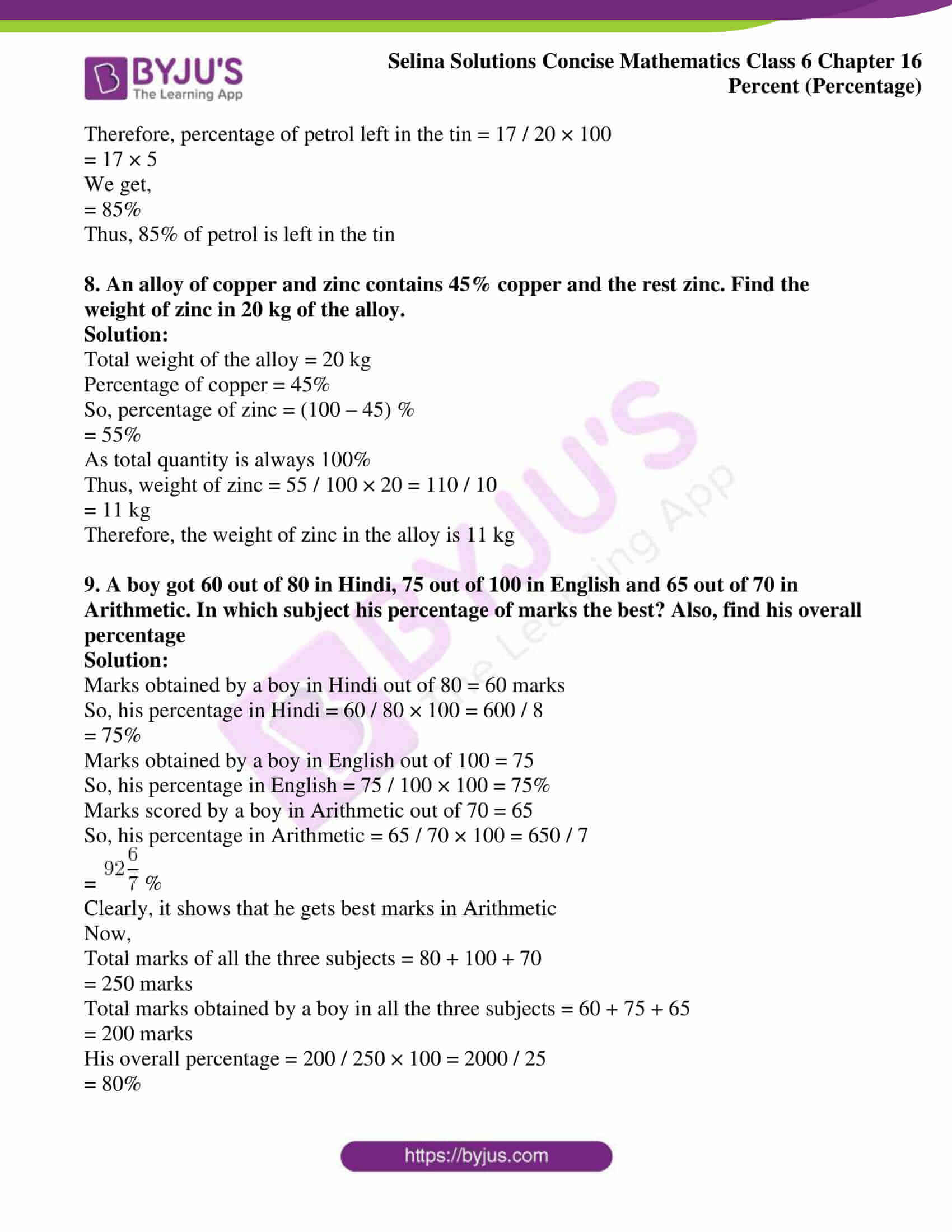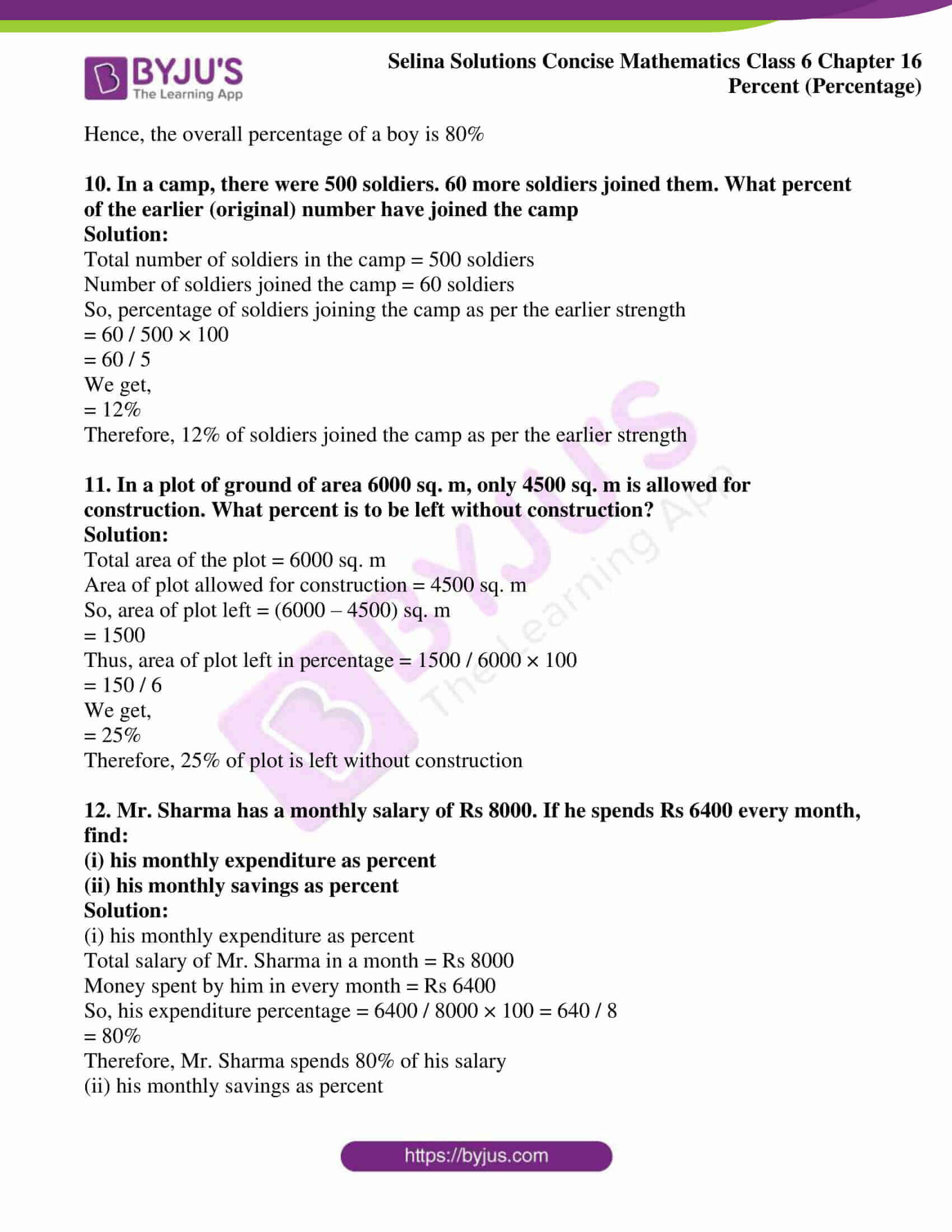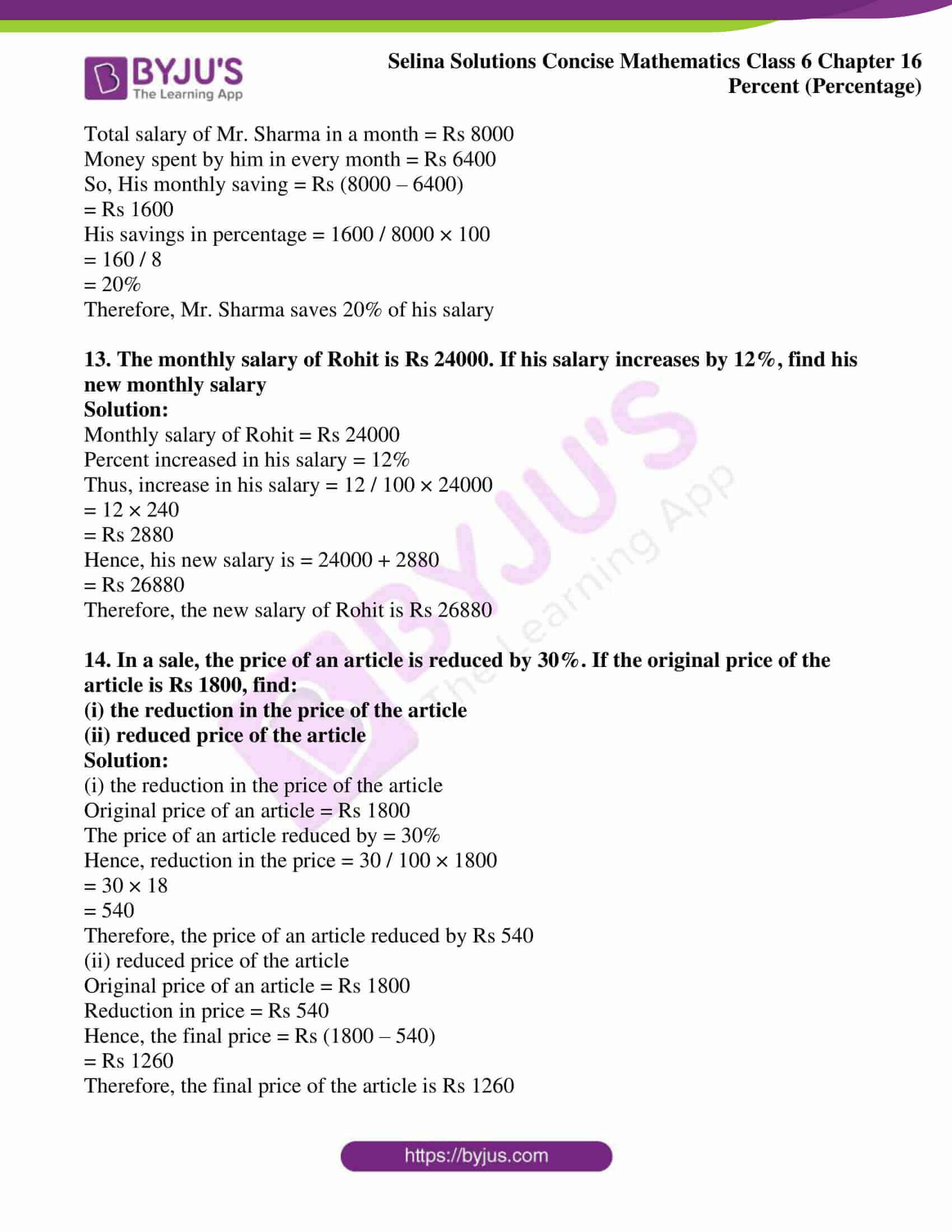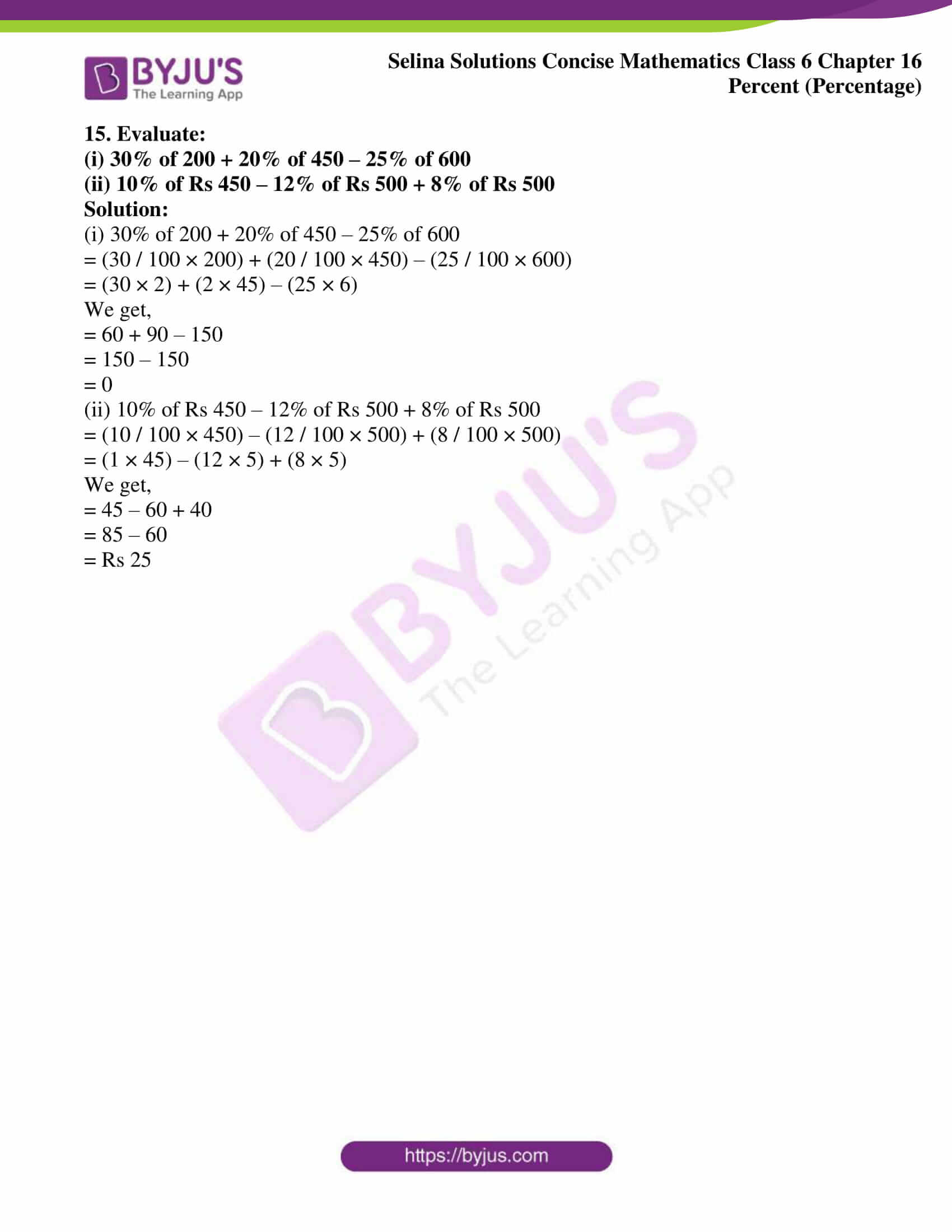### Access Selina Solutions Concise Mathematics Class 6 Chapter 16: Percent (Percentage) Exercise 16(B)

1. Express

(i) Rs 5 as a percentage of Rs 25

(ii) 80 paise as a percent of Rs 4

(iii) 700 gm as a percentage of 2.8 kg

(iv) 90 cm as a percent of 4.5 m

Solution:

(i) Rs 5 as a percentage of Rs 25

Rs 5 as a percentage of Rs 25 is given below

5 / 25 × 100

= 5 × 4

= 20%

Hence, Rs 5 is 20% of Rs 25

(ii) 80 paise as a percent of Rs 4

80 paise as a percent of Rs 4 is given below

We know that,

Rs 4 = 400 paisa

80 / 400 × 100

= 80 / 4

= 20%

Hence, 80 paisa is 20% of Rs 4

(iii) 700 gm as a percentage of 2.8 kg

700 gm as a percent of 2.8 kg is given below

We know that,

1 kg = 1000 gm

Thus, 2.8 kg = 2800 gm

So,

700 / 2800 × 100 = 700 / 28

= 100 / 4

We get,

= 25%

Hence, 700 gm is 25% of 2.8 kg

(iv) 90 cm as a percent of 4.5 m

90 cm as a percent of 4.5 m is given below

We know that,

1 m = 100 cm

Thus, 4.5 m = 450 cm

So,

90 / 450 × 100 = 900 / 45

= 20%

Hence, 90 cm is 20% of 4.5 m

2. Express the first quantity as a percent of the second:

(i) 40 P, Rs 2

(ii) 500 gm, 6 kg

(iii) 42 seconds, 6 minutes

Solution:

(i) 40 P, Rs 2

40 P as a percent of Rs 2 is shown below

We know that,

1 Rs = 100 P

So, 2 Rs = 200 P

40 / 200 × 100 = 40 / 2

We get,

= 20%

Hence, 40 P is 20 % of Rs 2

(ii) 500 gm, 6 kg

500 gm as a percent of 6 kg is shown below

We know that,

1 kg = 1000 gm

So, 6 kg = 6000 gm

500 / 6000 × 100 = 500 / 60

= 50 / 6

We get,

= 25 / 3

=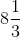%

Hence, 500 gm is% of 6 kg

(iii) 42 seconds 6 minutes

42 seconds as a percent of 6 minutes is shown below

We know that,

1 min = 60 sec

So, 6 min = 360 sec

42 / 360 × 100 = 420 / 36

= 35 / 3

We get,

=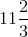%

Hence, 42 sec is% of 6 minutes

3. Find the value of each of the following:

(i) 20% of Rs 150

(ii) 90% of 130

(iii) 15% of 2 minutes

(iv) 7.5% of 500 kg

Solution:

(i) 20% of Rs 150

20% of Rs 150 is given below

20 / 100 × 150 = 2 × 15

We get,

= 30

Hence, 20% of Rs 150 is Rs 30

(ii) 90% of 130

90% of 130 is given below

90 / 100 × 130 = 9 × 13

We get,

= 117

Hence, 90% of 130 is 117

(iii) 15% of 2 minutes

15% of 2 minutes is given below

We know that,

1 min = 60 sec

So, 2 min = 120 sec

15 / 100 × 120 = (15 × 12) / 10

We get,

= 180 / 10

= 18 sec

Hence, 15% of 2 min is 18 sec

(iv) 7.5% of 500 kg

7.5% of 500 kg is given below

7.5 / 100 × 500 = 7.5 × 5

We get,

= 37.5 kg

Hence, 7.5% of 500 kg is 37.5 kg

4. If a man spends 70% of his income, what percent does he save?

Solution:

Let the total income of the man be = Rs 100

If a man spends 70% of his income, then total expense = 70 / 100 × 100

= Rs 70

Savings after his expenditure = 100 – 70 = 30

Hence, his savings in percent = 30 / 100 × 100

= 30%

Therefore, the man saved 30% of his total income

5. A girl gets 65 marks out of 80. What percent marks did she get?

Solution:

Total marks in the exam = 80

Marks obtained by a girl = 65

Her percentage = 65 / 80 × 100

= 650 / 8

We get,

= 81.25%

Therefore, the marks obtained by a girl in percentage is 81.25%

6. A class contains 25 children, of which 6 are girls. What percentage of the class are the boys

Solution:

Total number of children in the class = 25

Number of girls = 6

Number of boys = 25 – 6

= 19 boys

Thus, percentage of boys in the class = 19 / 25 × 100

= 19 × 4

We get,

= 76%

Therefore, there are 76% of boys in the class

7. A tin contains 20 litres of petrol. Due to leakage, 3 litres of petrol is lost. What percent is still present in the tin?

Solution:

Total quantity of petrol in the tin = 20 litres

Quantity of petrol lost, due to leakage = 3 litres

Now, quantity of petrol left in the tin = (20 – 3) = 17 litres

Therefore, percentage of petrol left in the tin = 17 / 20 × 100

= 17 × 5

We get,

= 85%

Thus, 85% of petrol is left in the tin

8. An alloy of copper and zinc contains 45% copper and the rest zinc. Find the weight of zinc in 20 kg of the alloy.

Solution:

Total weight of the alloy = 20 kg

Percentage of copper = 45%

So, percentage of zinc = (100 – 45) %

= 55%

As total quantity is always 100%

Thus, weight of zinc = 55 / 100 × 20 = 110 / 10

= 11 kg

Therefore, the weight of zinc in the alloy is 11 kg

9. A boy got 60 out of 80 in Hindi, 75 out of 100 in English and 65 out of 70 in Arithmetic. In which subject his percentage of marks the best? Also, find his overall percentage

Solution:

Marks obtained by a boy in Hindi out of 80 = 60 marks

So, his percentage in Hindi = 60 / 80 × 100 = 600 / 8

= 75%

Marks obtained by a boy in English out of 100 = 75

So, his percentage in English = 75 / 100 × 100 = 75%

Marks scored by a boy in Arithmetic out of 70 = 65

So, his percentage in Arithmetic = 65 / 70 × 100 = 650 / 7

=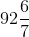%

Clearly, it shows that he gets best marks in Arithmetic

Now,

Total marks of all the three subjects = 80 + 100 + 70

= 250 marks

Total marks obtained by a boy in all the three subjects = 60 + 75 + 65

= 200 marks

His overall percentage = 200 / 250 × 100 = 2000 / 25

= 80%

Hence, the overall percentage of a boy is 80%

10. In a camp, there were 500 soldiers. 60 more soldiers joined them. What percent of the earlier (original) number have joined the camp

Solution:

Total number of soldiers in the camp = 500 soldiers

Number of soldiers joined the camp = 60 soldiers

So, percentage of soldiers joining the camp as per the earlier strength

= 60 / 500 × 100

= 60 / 5

We get,

= 12%

Therefore, 12% of soldiers joined the camp as per the earlier strength

11. In a plot of ground of area 6000 sq. m, only 4500 sq. m is allowed for construction. What percent is to be left without construction?

Solution:

Total area of the plot = 6000 sq. m

Area of plot allowed for construction = 4500 sq. m

So, area of plot left = (6000 – 4500) sq. m

= 1500

Thus, area of plot left in percentage = 1500 / 6000 × 100

= 150 / 6

We get,

= 25%

Therefore, 25% of plot is left without construction

12. Mr. Sharma has a monthly salary of Rs 8000. If he spends Rs 6400 every month, find:

(i) his monthly expenditure as percent

(ii) his monthly savings as percent

Solution:

(i) his monthly expenditure as percent

Total salary of Mr. Sharma in a month = Rs 8000

Money spent by him in every month = Rs 6400

So, his expenditure percentage = 6400 / 8000 × 100 = 640 / 8

= 80%

Therefore, Mr. Sharma spends 80% of his salary

(ii) his monthly savings as percent

Total salary of Mr. Sharma in a month = Rs 8000

Money spent by him in every month = Rs 6400

So, His monthly saving = Rs (8000 – 6400)

= Rs 1600

His savings in percentage = 1600 / 8000 × 100

= 160 / 8

= 20%

Therefore, Mr. Sharma saves 20% of his salary

13. The monthly salary of Rohit is Rs 24000. If his salary increases by 12%, find his new monthly salary

Solution:

Monthly salary of Rohit = Rs 24000

Percent increased in his salary = 12%

Thus, increase in his salary = 12 / 100 × 24000

= 12 × 240

= Rs 2880

Hence, his new salary is = 24000 + 2880

= Rs 26880

Therefore, the new salary of Rohit is Rs 26880

14. In a sale, the price of an article is reduced by 30%. If the original price of the article is Rs 1800, find:

(i) the reduction in the price of the article

(ii) reduced price of the article

Solution:

(i) the reduction in the price of the article

Original price of an article = Rs 1800

The price of an article reduced by = 30%

Hence, reduction in the price = 30 / 100 × 1800

= 30 × 18

= 540

Therefore, the price of an article reduced by Rs 540

(ii) reduced price of the article

Original price of an article = Rs 1800

Reduction in price = Rs 540

Hence, the final price = Rs (1800 – 540)

= Rs 1260

Therefore, the final price of the article is Rs 1260

15. Evaluate:

(i) 30% of 200 + 20% of 450 – 25% of 600

(ii) 10% of Rs 450 – 12% of Rs 500 + 8% of Rs 500

Solution:

(i) 30% of 200 + 20% of 450 – 25% of 600

= (30 / 100 × 200) + (20 / 100 × 450) – (25 / 100 × 600)

= (30 × 2) + (2 × 45) – (25 × 6)

We get,

= 60 + 90 – 150

= 150 – 150

= 0

(ii) 10% of Rs 450 – 12% of Rs 500 + 8% of Rs 500

= (10 / 100 × 450) – (12 / 100 × 500) + (8 / 100 × 500)

= (1 × 45) – (12 × 5) + (8 × 5)

We get,

= 45 – 60 + 40

= 85 – 60

= Rs 25